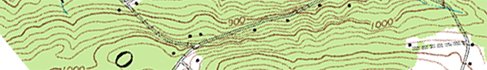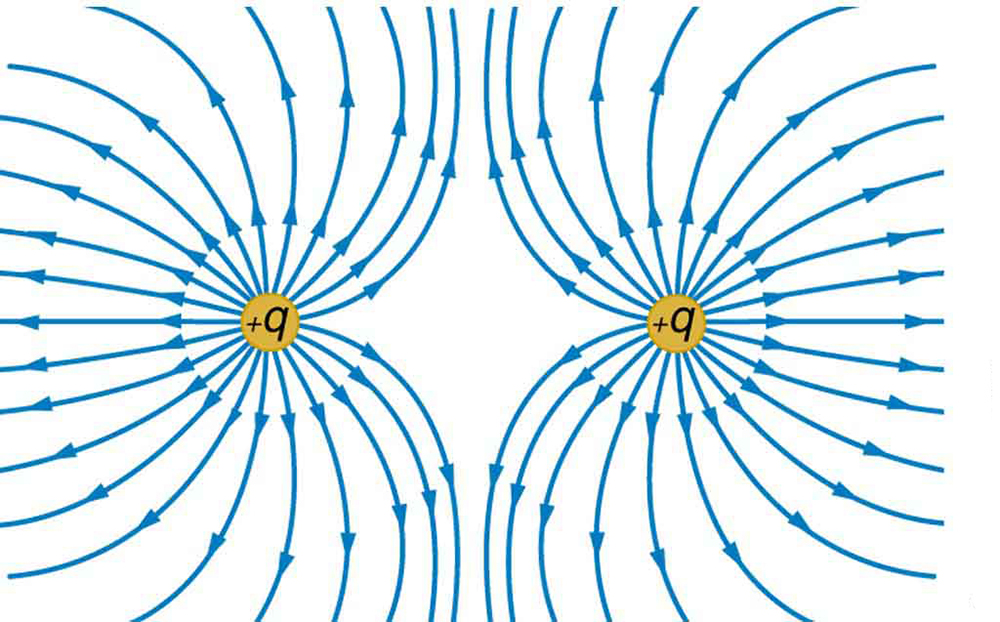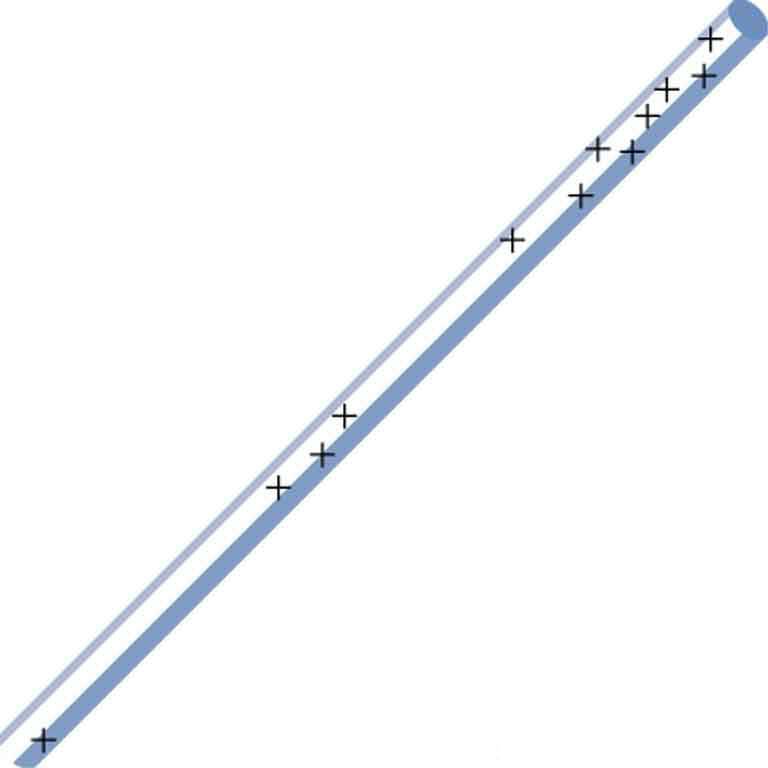# 19.4 Equipotential lines  (Page 3/5)

 Page 3 / 5

## Making connections: slopes and parallel plates

Consider the parallel plates in [link] . These have equipotential lines that are parallel to the plates in the space between, and evenly spaced. An example of this (with sample values) is given in [link] . One could draw a similar set of equipotential isolines for gravity on the hill shown in [link] . If the hill has any extent at the same slope, the isolines along that extent would be parallel to each other. Furthermore, in regions of constant slope, the isolines would be evenly spaced.Note that a topographical map along a ridge has roughly parallel elevation lines, similar to the equipotential lines in [link] .

An important application of electric fields and equipotential lines involves the heart. The heart relies on electrical signals to maintain its rhythm. The movement of electrical signals causes the chambers of the heart to contract and relax. When a person has a heart attack, the movement of these electrical signals may be disturbed. An artificial pacemaker and a defibrillator can be used to initiate the rhythm of electrical signals. The equipotential lines around the heart, the thoracic region, and the axis of the heart are useful ways of monitoring the structure and functions of the heart. An electrocardiogram (ECG) measures the small electric signals being generated during the activity of the heart. More about the relationship between electric fields and the heart is discussed in Energy Stored in Capacitors .

## Phet explorations: charges and fields

Move point charges around on the playing field and then view the electric field, voltages, equipotential lines, and more. It's colorful, it's dynamic, it's free.

## Test prep for ap courses

How would [link] be different with two positive charges replacing the two negative charges?

1. The equipotential lines would have positive values.
2. It would actually resemble [link] .
3. no change
4. not enough information

(a)

Consider two conducting plates, placed on adjacent sides of a square, but with a 1-m space between the corner of the square and the plate. These plates are not touching, not centered on each other, but are at right angles. Each plate is 1 m wide. If the plates are held at a fixed potential difference Δ V , draw the equipotential lines for this system.

As isolines of electric potential get closer together, the electric field gets stronger. What shape would a hill have as the isolines of gravitational potential get closer together?

1. constant slope
2. steeper slope
3. shallower slope
4. a U-shape

(b)

Between [link] and [link] , which more closely resembles the gravitational field between two equal masses, and why?

How much work is necessary to keep a positive point charge in orbit around a negative point charge?

1. A lot; this system is unstable.
2. Just a little; the isolines are far enough apart that crossing them doesn’t take much work.
3. None; we’re traveling along an isoline, which requires no work.
4. There’s not enough information to tell.

(c)

Consider two conducting plates, placed on adjacent sides of a square, but with a 1-m space between the corner of the square and the plate. These plates are not touching, not centered on each other, but are at right angles. Each plate is 1 m wide. If the plates are held at a fixed potential difference Δ V , sketch the path of both a positively charged object placed between the near ends, and a negatively charged object placed near the open ends.

## Section summary

• An equipotential line is a line along which the electric potential is constant.
• An equipotential surface is a three-dimensional version of equipotential lines.
• Equipotential lines are always perpendicular to electric field lines.
• The process by which a conductor can be fixed at zero volts by connecting it to the earth with a good conductor is called grounding.

## Conceptual questions

What is an equipotential line? What is an equipotential surface?

Explain in your own words why equipotential lines and surfaces must be perpendicular to electric field lines.

Can different equipotential lines cross? Explain.

## Problems&Exercises

(a) Sketch the equipotential lines near a point charge + $q$ . Indicate the direction of increasing potential. (b) Do the same for a point charge $–3\phantom{\rule{0.25em}{0ex}}q$ .

Sketch the equipotential lines for the two equal positive charges shown in [link] . Indicate the direction of increasing potential.The electric field near two equal positive charges is directed away from each of the charges.

[link] shows the electric field lines near two charges ${q}_{1}$ and ${q}_{2}$ , the first having a magnitude four times that of the second. Sketch the equipotential lines for these two charges, and indicate the direction of increasing potential.

Sketch the equipotential lines a long distance from the charges shown in [link] . Indicate the direction of increasing potential.

Sketch the equipotential lines in the vicinity of two opposite charges, where the negative charge is three times as great in magnitude as the positive. See [link] for a similar situation. Indicate the direction of increasing potential.

Sketch the equipotential lines in the vicinity of the negatively charged conductor in [link] . How will these equipotentials look a long distance from the object?

Sketch the equipotential lines surrounding the two conducting plates shown in [link] , given the top plate is positive and the bottom plate has an equal amount of negative charge. Be certain to indicate the distribution of charge on the plates. Is the field strongest where the plates are closest? Why should it be?

(a) Sketch the electric field lines in the vicinity of the charged insulator in [link] . Note its non-uniform charge distribution. (b) Sketch equipotential lines surrounding the insulator. Indicate the direction of increasing potential.A charged insulating rod such as might be used in a classroom demonstration.

The naturally occurring charge on the ground on a fine day out in the open country is $\text{–1}\text{.}\text{00}\phantom{\rule{0.25em}{0ex}}{\text{nC/m}}^{2}$ . (a) What is the electric field relative to ground at a height of 3.00 m? (b) Calculate the electric potential at this height. (c) Sketch electric field and equipotential lines for this scenario.

The lesser electric ray ( Narcine bancroftii ) maintains an incredible charge on its head and a charge equal in magnitude but opposite in sign on its tail ( [link] ). (a) Sketch the equipotential lines surrounding the ray. (b) Sketch the equipotentials when the ray is near a ship with a conducting surface. (c) How could this charge distribution be of use to the ray?Lesser electric ray ( Narcine bancroftii ) (credit: National Oceanic and Atmospheric Administration, NOAA's Fisheries Collection).

Why does earth exert only a tiny downward pull?
hello
Islam
Why is light bright?
an 8.0 capacitor is connected by to the terminals of 60Hz whoes rms voltage is 150v. a.find the capacity reactance and rms to the circuit
thanks so much. i undersooth well
what is physics
is the study of matter in relation to energy
Kintu
a submersible pump is dropped a borehole and hits the level of water at the bottom of the borehole 5 seconds later.determine the level of water in the borehole
what is power?
power P = Work done per second W/ t. It means the more power, the stronger machine
Sphere
e.g. heart Uses 2 W per beat.
Rohit
A spherica, concave shaving mirror has a radius of curvature of 32 cm .what is the magnification of a persons face. when it is 12cm to the left of the vertex of the mirror
did you solve?
Shii
1.75cm
Ridwan
my name is Abu m.konnek I am a student of a electrical engineer and I want you to help me
Abu
the magnification k = f/(f-d) with focus f = R/2 =16 cm; d =12 cm k = 16/4 =4
Sphere
what do we call velocity
Kings
A weather vane is some sort of directional arrow parallel to the ground that may rotate freely in a horizontal plane. A typical weather vane has a large cross-sectional area perpendicular to the direction the arrow is pointing, like a “One Way” street sign. The purpose of the weather vane is to indicate the direction of the wind. As wind blows pa
hi
Godfred
Godfred
If a prism is fully imersed in water then the ray of light will normally dispersed or their is any difference?
the same behavior thru the prism out or in water bud abbot
Ju
If this will experimented with a hollow(vaccum) prism in water then what will be result ?
Anurag
What was the previous far point of a patient who had laser correction that reduced the power of her eye by 7.00 D, producing a normal distant vision power of 50.0 D for her?
What is the far point of a person whose eyes have a relaxed power of 50.5 D?
Jaydie
What is the far point of a person whose eyes have a relaxed power of 50.5 D?
Jaydie
A young woman with normal distant vision has a 10.0% ability to accommodate (that is, increase) the power of her eyes. What is the closest object she can see clearly?
Jaydie
29/20 ? maybes
Ju
In what ways does physics affect the society both positively or negatively
how can I read physics...am finding it difficult to understand...pls help
try to read several books on phy don't just rely one. some authors explain better than other.
Ju
And don't forget to check out YouTube videos on the subject. Videos offer a different visual way to learn easier.
Ju
hope that helps
Ju
I have a exam on 12 february
what is velocity
Jiti
the speed of something in a given direction.
JuByByBy